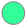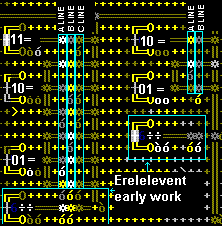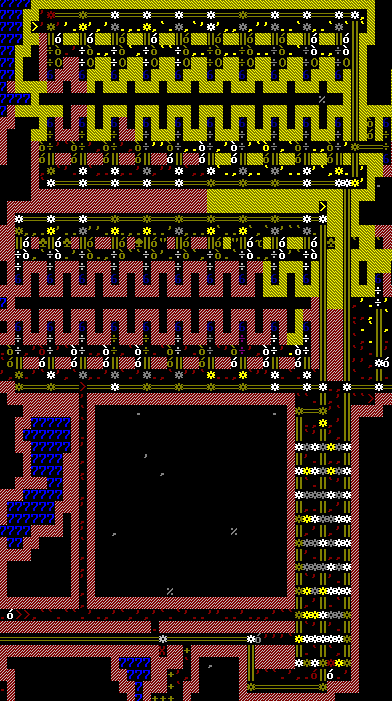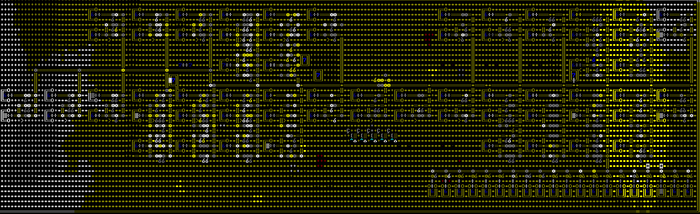• v50 information can now be added to pages in the main namespace. v0.47 information can still be found in the DF2014 namespace. See here for more details on the new versioning policy.
• Use this page to report any issues related to the migration.
This notice may be cached—the current version can be found here.

# User:BaronW

I built Space invaders into Dwarf Fortress.

It can be downloaded From here The map can be seen here You can see a video of a play-through here

I will update this with details of how I made the project as I have time.

### Stats

This fort took 85000 mechanisms. It takes over 100,000 power at full drain. The main screen is 32x32 that makes it a 1 kilopixel display.

### High Level Overview

Sorry this is so big

### More Detail

#### KEYGear assembly where the starting position is engagedGear assembly where the starting position is disengagedPressure plate switch: the letter in the boxes indicates the type of switch C is Newton's Cradle S is Power to Signal. The Symbol in the dimond matches linked Items.

## The Almighty Dwarven Calculator

What can you do with 75,368 mechanisms and 40,000 power?
I have made a calculator. It can add, subtract, divide and multiply. It can take inputs of up to 999 and give answers of up to 999999 or 999.999.

See the map - 
Download the fort -  There are probably still several bugs, if you find one list it on the discussion page.

### How to use

In the control house (shown above calculating 7x191) there 6 groups of levers each group corresponds to an input number, and each lever within a group corresponds to a number 0-9 (in the same pattern as on a cell phone, as shown below).

Once the levers that represent the desired numbers have been pulled (only one lever per group or things will go wrong) then select the desired function (+ * - /) by pulling the indicated lever. The selected function will be displayed.

Then pull the equals lever, go and make a cup of tea, some soup perhaps compose a sonnet (it takes a long time even for simple equations).

Your answer will then be displayed in glorious digital numbers (many incorrect numbers will 'flash past' first once the numbers stop changing the answer will be correct [I think])

### How it works

In essence what you need to do to make any computer work is to break the job down to its simplest possible functions. To that end all actual computation is done in binary.
This is the first 2 cells from the addition engine.Each cell is designed to add a two or three binary digits together. The first cell adds 2 digits (that is one from each of the input numbers). When you add 2 binary digits together there are 4 possible ways for them to combine and 3 possible outcomes (0+0=0, 0+1=1, 1+0=1, 1+1=10), however an outcome of 0 does not change the answer so you do not need to build it (In the addition engine I did build it but I later disconnected it). So each input gets a line of mechanisms, A line and B line, since there are 3 important ways of combining the inputs each line has 3 mechanisms. levers are connected to some mechanisms so they can be 'flipped' and thereby each row made to represent one possible outcome. A screw-pump is then connected to each outcome which triggers a pressure-plate which triggers either the outcome or the C line of the next cell.
The second and all subsequent cells need to add a C line that is triggered when the answer of the previous cell is 10 or 11. And so there becomes 8 (7 important) ways for the digits to combine and 4 possible outcomes (3 important). But an all other ways cell 2-10 is the same as cell 1.And the whole addition engine looks like this#### Subtraction (binary)

Subtraction works much the same as addition except that the A-line input is positive and the B-line and C-line inputs are negative. So the possible outcomes are (from a 3 line input) (in the order I put them in the engine) 1-0-0=01, 1-1-1=-11, 0-0-1=-11, 0-1-0=-11, 0-1-1=-10, 1-0-1=0, 1-1-0=0, 0-0-0=0. This has the advantage that there are 3 irrelevant outcomes in each cell so you only need to build 5 rows in each cell.
Subtraction engine.The one difficulty in subtraction is that the engine has no idea what a negative number is, so if you want the calculator to be able to give a negative response you need to teach it. My solution (possibly massively overcomplicated) was to build a 'which number is larger' engine and then tell the subtraction engine to subtract the smaller number from the larger. A 'which number is larger' engine is not easy. It is presented with 2 10 digit binary numbers A1-A10 & B1-B10 then asks: does 'A1=1 and B1=0' or does 'B1=1 n and A1=0' if the answer is yes to either, that will mean that one number is larger than the other. It will then send power to a set of screw-pumps that will then tell the main engine which number to subtract from which. If the answer is no to both then it will ask does 'A2=1 and B2=0' or does 'B2=1 n and A2=0', this repeats until it decided which number is larger or that they are the same. I am sure there are better ways of doing this but I was to tired at the time to think of them.#### Multiplication (binary)

Multiplication works almost exactly like adding another dimension to addition. To multiply 2 numbers together we once again need to break the process down. So in decimal 238x644 = 238x4+238x40+238x600. The same is true in binary except the whole thing is much easier because you are either multiplying by 1 or 0. So 101x110 breaks down to 101x0+101x10+101x100 simple. Once you realise this it is easy design the multiplication engine (rather harder to build)it is simply a series of addition engines or tiers where the A-line of each cell is either the A-number or 0 depending on the B-number and the B-line is the answer from the tier above (shifted 1 to the left so as to effectively multiply it by 10).

#### Division (binary)

Division is where it gets absurd. It is somewhat like adding another dimension to subtraction, but more. When you do long division by hand you break down the operation into small simple divisions. First you divide the first digit of the dividend by the divisor, you put the answer in the answer line and add any remainder to the remaining digits of the divisor. You then repeat this operation until there is no remaining dividend or you reach the desired number of decimal places. Again the nature of binary makes this very simple if the divisor is smaller, then the answer line gets a 1 and the divisor is subtracted from the segment of the dividend it was being compared to. If the divisor is larger then the answer line gets a 0 and the operation moves on one step. In dwarven engineering terms it looks like this.

#### Translation (Decimal to Binary)

Actually very easy each input number simply needs to be broken down to its individual digits these digits are then translated to binary and added together. Looks like this.#### Translation (Binary to Decimal)

This is harder. For 3 reasons. First programing in decimal would be to absurdly complex even for this fort, so simply adding the numbers together is out. Second 999x999= a six digit number. Third for some god forsaken reason I was determined to make it accurate to 3 decimal places so I needed to build a separate engine capable of handling decimal places. The only way I could figure to make this work is for the engine to ask - is the answer larger than or equal to 900,000 (if yes subtract 900,000 from the answer and move to the 10,000's line) if no is the answer larger than or equal to 800,000 -- repeat until you reach - is the answer larger than 1. That is 54 operations. Do not try this at home.